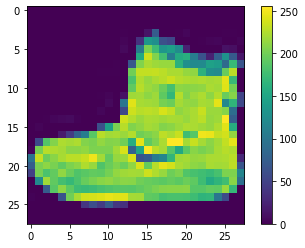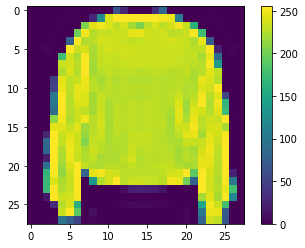# Progress Update 1

Workshop Resources

# Importing TensorFlow and tf.keras libraries
import tensorflow as tf
from tensorflow import keras

# Helper libraries for statistics and plotting
import numpy as np
import matplotlib.pyplot as plt

#This variable is declared from the fashion_mist library of the datasets section
fashion_mnist = keras.datasets.fashion_mnist

#This loads four variables from the dataset.
#The train_images and train_labels are data that the model uses to learn
#The test_images and test_labels are used by the model to compare against.
(train_images, train_labels), (test_images, test_labels) = fashion_mnist.load_data()

class_names = ['T-shirt/top', 'Trouser', 'Pullover', 'Dress', 'Coat', 'Sandal', 'Shirt', 'Sneaker', 'Bag', 'Ankle boot'  ]

plt.figure()
plt.imshow(train_images) #Shows the first image in the data set as a plot or different colored pixels
plt.colorbar() #displays the color bar on the right
plt.grid(False)
plt.show() #displays the entire plotplt.figure()
plt.imshow(train_images) #Shows the first image in the data set as a plot or different colored pixels
plt.colorbar() #displays the color bar on the right
plt.grid(False)
plt.show() #displays the entire plot#the train_images and test_images range between values from 0 to 255.
#To maintain consistency between the training and testing set, we will divide train_images and test_images by 255
train_images = train_images / 255.0
test_images = test_images / 255.0